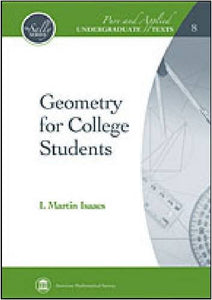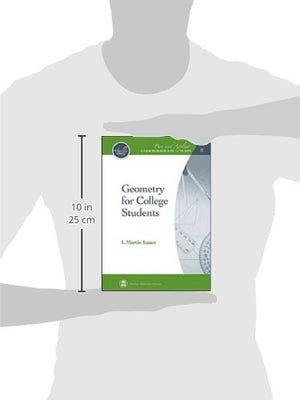Geometry for College Students (Pure and Applied Undergraduate Texts)

• Publish Date: 2009-03-10
• Binding: Hardcover
• Author: I. Martin Isaacs
Regular price \$72.54 Sale price \$40.66

One of the challenges many mathematics students face occurs after they complete their study of basic calculus and linear algebra, and they start taking courses where they are expected to write proofs. Historically, students have been learning to think mathematically and to write proofs by studying Euclidean geometry. In the author's opinion, geometry is still the best way to make the transition from elementary to advanced mathematics. The book begins with a thorough review of high school geometry, then goes on to discuss special points associated with triangles, circles and certain associated lines, Ceva's theorem, vector techniques of proof, and compass-and-straightedge constructions. There is also some emphasis on proving numerical formulas like the laws of sines, cosines, and tangents, Stewart's theorem, Ptolemy's theorem, and the area formula of Heron. An important difference of this book from the majority of modern college geometry texts is that it avoids axiomatics. The students using this book have had very little experience with formal mathematics. Instead, the focus of the course and the book is on interesting theorems and on the techniques that can be used to prove them. This makes the book suitable to second- or third-year mathematics majors and also to secondary mathematics education majors, allowing the students to learn how to write proofs of mathematical results and, at the end, showing them what mathematics is really all about.